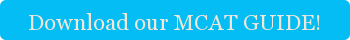I know this will blow your mind, but I have to tell you something: modern medicine has yet to discover the healing potential of pendulums, or the prophylactic power of masses on springs. Yet hope “springs” eternal, I suppose. Certainly, after years as an MCAT tutor, I can’t fathom any other reason beside Hope why the MCAT insists on torturing poor doctors-to-be with contrived situations involving both of these elements. But alas, it does.  The MCAT coach doesn’t pick the fight; I just get you ready to step in the ring. Such is life—such, in a way, is medicine.  Maybe that’s the point.

So what are the best MCAT study tips to deal with these esoteric problems? There are two. The first is an intuitive understanding of two questions: which variables affect the motion and how those variables will affect the motion. The second is an appreciation of the symmetry between both the periodic motion formulas for pendulums and masses on springs, which might help you remember the formulas (you should remember the formulas).

First, commonalities. Both types of periodic motion depend on some sort of restoring force. The restoring force attempts to bring the mass (the weight on the end of the pendulum or the mass on the spring) back some neutral point. The neutral point for a pendulum is when it is hanging straight down vertically, and the neutral point for a mass on a spring is when the spring is not at all deformed. Hence, the restoring force for a pendulum is gravitational, and the restoring force for a mass on a spring is the force exerted by a deformed spring.

For a pendulum, a great restoring force (more gravity), results in a shorter period. This makes sense; things fall faster when there is more gravity, and the mass on the pendulum is no exception. Alternatively, the period of a pendulum gets longer when the length of the arm is longer. The longer the arm of the pendulum, the longer the arc the pendulum has to travel to complete a period. The formula for the period of a pendulum can be summarized:

T=2π*√(l/g)

where T is the period, l is the length of the pendulum and g is the force of gravity. Notice what it doesn’t include; the mass at the end of the pendulum does not affect its period!

Now consider the mass on a spring. Making the mass heavier increases the force necessary to accelerate it, so a larger mass has a longer period. On the other hand, a spring with a larger spring constant generates more force at a given deformity, so making the spring constant larger results in a shorter period. The formula for the period of a mass on a spring can be summarized:

T=2π*√(m/k)

where T is the period, m is the mass on the spring and k is the spring constant. Notice what it doesn’t include; the distance you stretch the spring doesn’t matter!

Now, observe the symmetry. They’re basically the same formula; just sub in m/k for l/g or vice versa! The best MCAT preparation involves making connections between different concepts to solidify your understanding. When studying for the MCAT, seek to form these connections for the most effective preparation.Tags: physics, MCAT